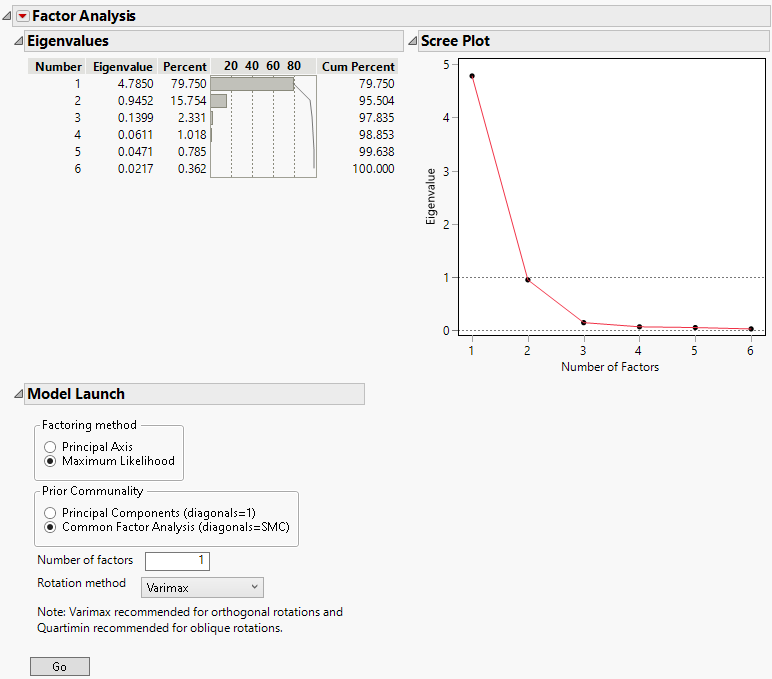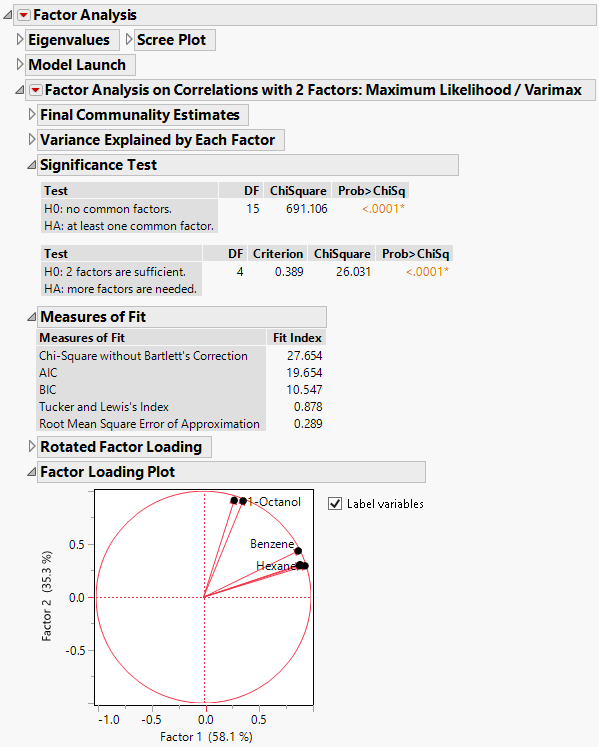Multivariate Methods > Factor Analysis > Example of the Factor Analysis Platform
Publication date: 11/10/2021

# Example of the Factor Analysis Platform

This example uses the Solubility.jmp sample data table to extract two factors explained by six solvents.

1. Select Help > Sample Data Library and open Solubility.jmp.

2. Select Analyze > Multivariate Methods > Factor Analysis.

3. Select 1-Octanol through Hexane and click Y, Columns.

4. Click OK.

Figure 9.2 Initial Factor Analysis ReportThe elbow on the scree plot indicates that a model with two factors is appropriate.

5. In the Model Launch outline, make the following selections:

Factoring Method= Maximum Likelihood

Prior Communality= Common Factor Analysis

Number of factors = 2

Rotation Method= Varimax

6. Click Go.

Figure 9.3 Factor Analysis ReportThe report lists the communality estimates, variance estimates, significance tests, measures of fit, rotated factor loadings, and a factor loading plot. The Rotated Factor Loadings and Factor Loading Plot suggest that Factor 1 relates to the Carbon Tetrachloride-Chloroform-Benzene-Hexane cluster of variables, and that Factor 2 relates to the Ether–1-Octanol cluster of variables. See Factor Analysis Model Fit Options for details of the information shown in the report.

Tip: Click the points in the Factor Loading Plot to select and move the labels. Click in the lower right corner to increase the plot size to more easily view the labels.